# HDL Optimizations Across MATLAB Function Block Boundary Using MATLAB Datapath Architecture

This example shows how to use various optimizations inside the MATLAB Function block and across the MATLAB Function block boundary with other blocks in your Simulink® model. The example also illustrates the difference in area and timing when you use different HDL architecture settings of the MATLAB Function block.

### Why Use MATLAB Datapath Architecture?

HDL code generation for a MATLAB Function block supports two HDL architectures: `MATLAB Function` and `MATLAB Datapath`. Specify the HDL Architecture in the HDL Block Properties dialog box of the MATLAB Function block.

Use the `MATLAB Datapath` architecture to;

• Model complex fixed-point and floating-point MATLAB algorithms inside MATLAB Function blocks and interface this algorithm with other Simulink blocks in your model.

• Improve area and timing of your design significantly by optimizing the algorithm inside the MATLAB Function block and across the MATLAB Function block boundary with other Simulink blocks in your model.

This architecture is the default setting for MATLAB Function blocks with floating-point types. By enabling the `MATLAB Datapath` architecture for fixed-point operations, you can use various optimizations that include:

• Hierarchy flattening

• Resource sharing and streaming

• Clock-rate pipelining

• Distributed pipelining and hierarchical distributed pipelining

• Critical path estimation

### How MATLAB Datapath Architecture Works

Fixed-point Simulink® models use the `MATLAB Function` architecture by default. Certain HDL optimizations such as resource sharing and distributed pipelining that you enable with this architecture optimize the blocks surrounding the MATLAB Function block and the algorithm inside the MATLAB Function block. To see the effect of the optimizations inside the MATLAB Function block, examine the generated HDL code for the block. This architecture does not apply the optimizations across the MATLAB Function block boundary with other Simulink blocks.

Floating-point Simulink models use the `MATLAB Datapath` architecture even if you specify `MATLAB Function` as the architecture setting for the block. When you use floating-point types, specify the native floating-point mode. With this architecture, the code generator treats the block like a regular Subsystem block. HDL Coder transforms the control flow algorithm of the MATLAB code inside the MATLAB Function block to a dataflow representation that uses Simulink blocks. The `MATLAB Datapath` architecture unrolls loops in your code due to this transformation. If you want to stream loops, either use the loop streaming optimization with the `MATLAB Function` architecture or use the streaming optimization with `MATLAB Datapath` as the HDL architecture.

By using the `MATLAB Datapath` architecture, You can more effectively perform various HDL Coder™ optimizations with the MATLAB Function block that you would otherwise perform with a Subsystem block. The `MATLAB Datapath` architecture applies the optimization settings that you specify on the algorithm inside the MATLAB Function block and across the MATLAB Function block boundary with other blocks in your Simulink model.

For example, consider this model with a DUT Subsystem that consists of a Product block and a MATLAB Function block.

```open_system('hdlcoder_MLFB_simple_datapath') set_param('hdlcoder_MLFB_simple_datapath', 'SimulationCommand', 'Update') open_system('hdlcoder_MLFB_simple_datapath/HDL_DUT') ```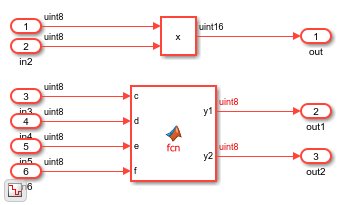The MATLAB Function block implements two multiplications.

```open_system('hdlcoder_MLFB_simple_datapath/HDL_DUT/MATLAB Function') ```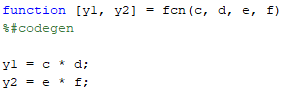The HDL architecture of the MATLAB Function block is set to `MATLAB Datapath`. To generate HDL code for the `HDL_DUT` Subsystem, run this command:

```makehdl('hdlcoder_MLFB_simple_datapath/HDL_DUT') ```

When you generate HDL code, the code generator replaces the MATLAB Function block with a subsystem that performs the multiplications `c * d` and `e * f`.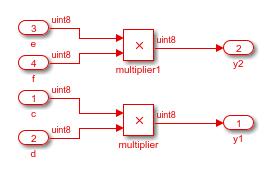With the `MATLAB Datapath` architecture, you can perform optimizations inside the MATLAB Function block and across the MATLAB Function block with other Simulink blocks. In this example, you can share the two multipliers inside the MATLAB Function block. To optimize the blocks, set the SharingFactor to `2` on the MATLAB Function block.

```mlsubsys = 'hdlcoder_MLFB_simple_datapath/HDL_DUT/MATLAB Function'; hdlset_param(mlsubsys, 'SharingFactor', 2) ```

When you generate HDL code, the code generator shares the multiplications inside the MATLAB Function block. The sharing group is displayed in the Optimization Report. When you click the links in the sharing group, HDL Coder displays the shared multipliers inside the MATLAB Function block in the generated model and the original model.You can apply the optimization across the MATLAB Function block with other Simulink blocks. In this example, you can share the Product block outside the MATLAB Function block with the multipliers inside the MATLAB Function block. To share these resources, remove the SharingFactor on the MATLAB Function block, and on the parent subsystem, `HDL_DUT`, enable FlattenHierarchy and set SharingFactor to `3`.

```hdlset_param(mlsubsys, 'SharingFactor', 0) hdlset_param('hdlcoder_MLFB_simple_datapath/HDL_DUT', ... 'FlattenHierarchy', 'on', 'SharingFactor', 3) ```

Note: Do not use the `InlineMATLABCode` property with the `MATLAB Datapath` architecture of the block. Use `FlattenHierarchy` instead.

When you generate HDL code, the code generator shares the multiplications inside the MATLAB Function block with the Product block outside. You see the sharing group of three multipliers in the Optimization Report. When you click the links in the sharing group, the shared multipliers are highlighted in the generated model and the original model.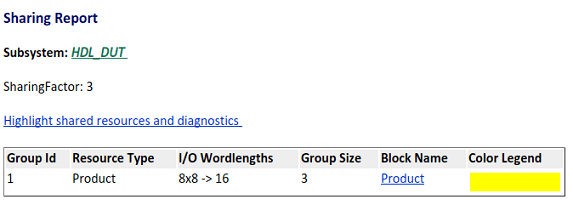### MATLAB Function Block Model With Default MATLAB Function Architecture

For an example model that illustrates the `MATLAB Datapath` architecture and how it differs from the `MATLAB Function` architecture, open the model `hdlcoder_MLFB_share_pipeline`. The model uses integer types. For an example that illustrates how you use the `MATLAB Datapath` architecture with floating-point types, see Generate Target-Independent HDL Code with Native Floating-Point.

```open_system('hdlcoder_MLFB_share_pipeline') set_param('hdlcoder_MLFB_share_pipeline','SimulationCommand','Update') ```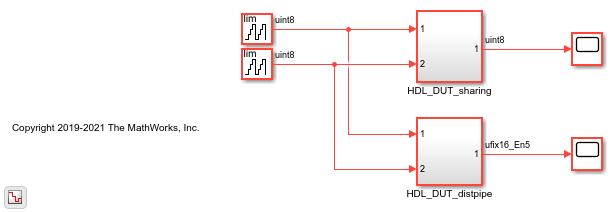The model contains two DUT subsystems at the top level `HDL_DUT_sharing` and `HDL_DUT_distpipe`. The subsystems illustrate how you can use resource sharing and distributed pipelining optimizations across the MATLAB Function block boundary with other blocks. Both subsystems perform basic additions and multiplications inside and outside the MATLAB Function block.

```open_system('hdlcoder_MLFB_share_pipeline/HDL_DUT_sharing') ```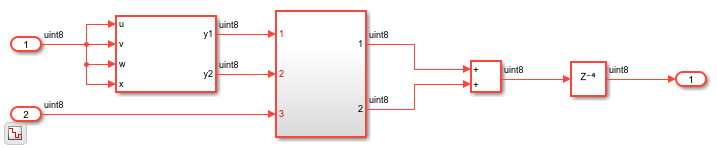```open_system('hdlcoder_MLFB_share_pipeline/HDL_DUT_sharing/Subsystem') ``````open_system('hdlcoder_MLFB_share_pipeline/HDL_DUT_distpipe') ```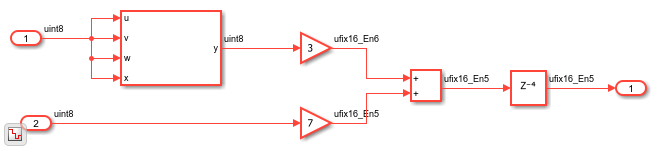To see the HDL parameters that are saved on the model, run the `hdlsaveparams` function.

```hdlsaveparams('hdlcoder_MLFB_share_pipeline') ```
```%% Set Model 'hdlcoder_MLFB_share_pipeline' HDL parameters hdlset_param('hdlcoder_MLFB_share_pipeline', 'CriticalPathEstimation', 'on'); hdlset_param('hdlcoder_MLFB_share_pipeline', 'HDLSubsystem', 'hdlcoder_MLFB_share_pipeline/HDL_DUT_sharing'); hdlset_param('hdlcoder_MLFB_share_pipeline', 'OptimizationReport', 'on'); hdlset_param('hdlcoder_MLFB_share_pipeline', 'Oversampling', 40); hdlset_param('hdlcoder_MLFB_share_pipeline', 'ResourceReport', 'on'); hdlset_param('hdlcoder_MLFB_share_pipeline', 'ShareAdders', 'on'); hdlset_param('hdlcoder_MLFB_share_pipeline', 'TargetDirectory', 'hdl_prj\hdlsrc'); hdlset_param('hdlcoder_MLFB_share_pipeline', 'Traceability', 'on'); % Set SubSystem HDL parameters hdlset_param('hdlcoder_MLFB_share_pipeline/HDL_DUT_distpipe', 'DistributedPipelining', 'on'); hdlset_param('hdlcoder_MLFB_share_pipeline/HDL_DUT_distpipe', 'FlattenHierarchy', 'on'); % Set SubSystem HDL parameters hdlset_param('hdlcoder_MLFB_share_pipeline/HDL_DUT_sharing', 'FlattenHierarchy', 'on'); hdlset_param('hdlcoder_MLFB_share_pipeline/HDL_DUT_sharing', 'SharingFactor', 8); ```

You see that the default `MATLAB Function` HDL architecture is saved on the model.

### Generate HDL Code using MATLAB Function Architecture

To generate HDL code for the sharing DUT, run this command:

```makehdl('hdlcoder_MLFB_share_pipeline/HDL_DUT_sharing') ```

When you open the Streaming and Sharing Report, the report displays four multipliers and three adders as shared resources.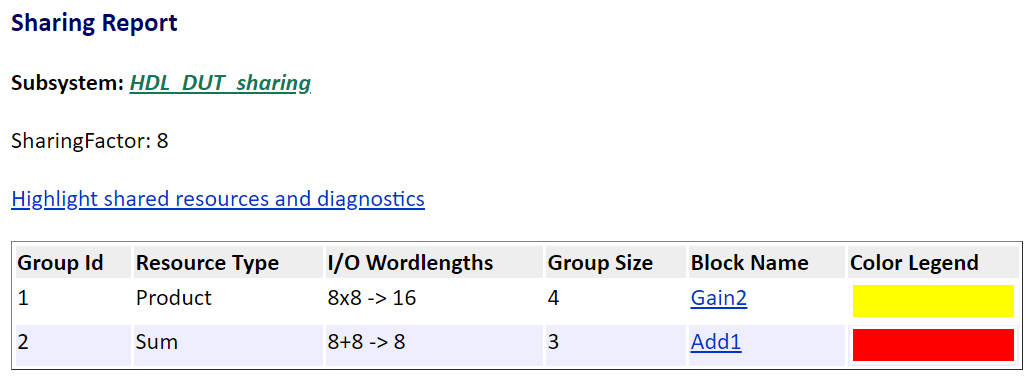When you click the second sharing group, the code generator highlights three adders surrounding the MATLAB Function block. The sharing group includes the two adders inside the Subsystem and the Add block outside. The code generator did not share the multipliers and adders that are inside the MATLAB Function block.

Critical path estimation is enabled on the model. When you annotate the critical path, the MATLAB Function block acts as a barrier to this optimization. If the critical path is inside the MATLAB Function block and if you want to highlight the critical path, use the `MATLAB Datapath` architecture.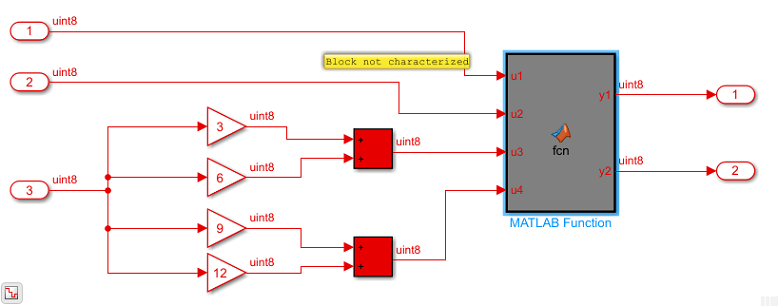To generate HDL code for `HDL_DUT_distpipe`, run this command:

```makehdl('hdlcoder_MLFB_share_pipeline/HDL_DUT_distpipe') ```

When you open the Distributed Pipelining Report, you see that the code generator moved pipelines inside the `HDL_DUT_distpipe` subsystem but did not distribute pipelines inside the MATLAB Function block.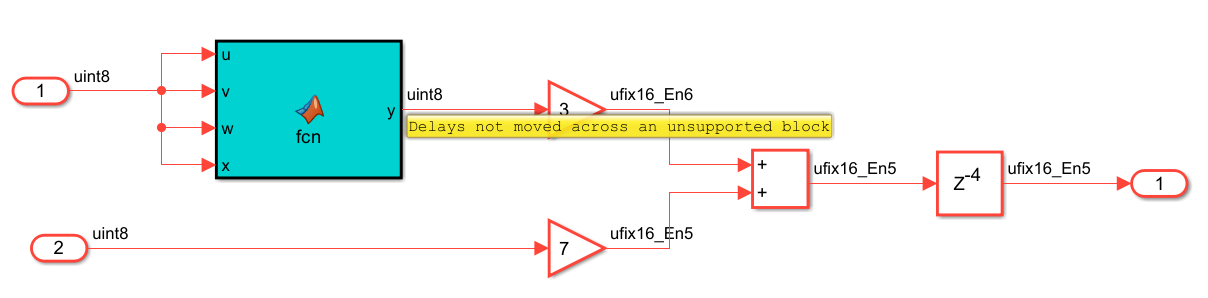This figure displays how distributed pipelining moved pipeline registers inside the subsystem.### Apply Optimizations Across MATLAB Function Block and Other Simulink Blocks

To improve area and timing of your design, use the `MATLAB Datapath` architecture. For the `HDL_DUT_sharing` subsystem, you can combine resource sharing with clock-rate pipelining and share the resources inside the MATLAB Function block and across the MATLAB Function block with other blocks.

To share resources:

1. Enable FlattenHierarchy and specify a SharingFactor on the parent subsystem `HDL_DUT_sharing`. Set the SharingFactor to `8`.

```share_subsys = 'hdlcoder_MLFB_share_pipeline/HDL_DUT_sharing'; hdlset_param(share_subsys, 'FlattenHierarchy', 'on', 'SharingFactor', 8); ```

2. Specify the `MATLAB Datapath` architecture for the MATLAB Function blocks inside the `HDL_DUT_sharing` subsystem.

```share_mlfcn1 = 'hdlcoder_MLFB_share_pipeline/HDL_DUT_sharing/MATLAB Function'; share_mlfcn2 = 'hdlcoder_MLFB_share_pipeline/HDL_DUT_sharing/Subsystem/MATLAB Function'; hdlset_param(share_mlfcn1, 'architecture', 'MATLAB Datapath'); hdlset_param(share_mlfcn2, 'architecture', 'MATLAB Datapath'); ```

When you open the Streaming and Sharing report, the report displays a sharing group of eight multipliers and two sharing groups of adders.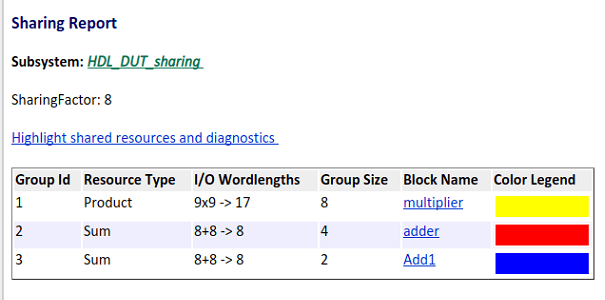When you select the first sharing group, you see that the optimization shared the multipliers inside the MATLAB Function with the four Gain blocks outside. The second sharing group consists of adders inside each of the two MATLAB Function blocks.

You can use the distributed pipelining optimization with the `Distributedpipe_MLFB` subsystem. Enable hierarchical distributed pipelining at the top level and set the HDL architecture of the MATLAB Function to `MATLAB Datapath` with DistributedPipelining set to `on`.

```hdlset_param('hdlcoder_MLFB_share_pipeline', 'HierarchicalDistPipelining', 'on'); dist_subsys = 'hdlcoder_MLFB_share_pipeline/HDL_DUT_distpipe/MATLAB Function'; hdlset_param(dist_subsys, 'architecture', 'MATLAB Datapath'); hdlset_param(dist_subsys, 'DistributedPipelining', 'on'); ```

After you generate HDL code, open the generated model. The code generator uses hierarchical distributed pipelining and distributed pipelining to move the pipeline registers across the blocks and inside the `MATLAB Function` Subsystem.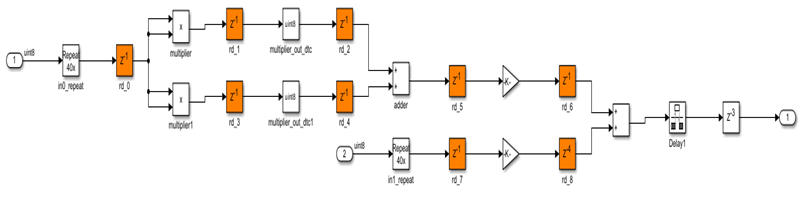This figure displays how distributed pipelining moved pipeline registers inside the `MATLAB Function` Subsystem.